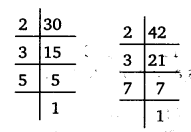# Class 6 Maths NCERT Solutions for Chapter 3 Playing with Numbers Ex 3.6

## Playing with Numbers

Question 1.
Find the HCF of the following numbers :
(a)
18, 48
(b) 30, 42
(c) 18, 60
(d) 27, 63
(e) 36, 84
(f) 34, 102
(g) 70, 105, 175
(h) 91, 112, 49
(i) 18, 54, 81
(j) 12, 45, 75

Solution:
(a) First, we write the prime factorisation of each of the given numbers. We have,∴ 18 = 2 x 3 x 3
and 48 = 2 x 2 x 2 x 2 x 3
We find that 2 and 3 each occurs as a common factor in the given numbers at least once.
∴ Required HCF = 2 x 3 = 6

(b) First, we write the prime factorisation of each of the given numbers.
We have,∴ 30 = 2 x 3 x 5
and 42 = 2 x 3 x 7
We find that 2 and 3 each occurs as a common factor in the given numbers at least once.
Required HCF = 2 x 3 = 6

(c) First, we write the prime factorisation of each of the given numbers.
We have,∴ 18 = 2 x 3 x 3
and 60 = 2 x 2 x 3 x 5
We find that 2 and 3 each occurs as a common factor in

(d) 27,63
Factors of 27 are 1, 3, 9 and 27.
Factors of 63 are 1, 3, 7, 9, 21 and 63.
Common factors of 27 and 63 are 1, 3 and 9.
Highest of these common factors is 9.
∴ H.C.F. of 27 and 63 is 9.

(e) 36,84
Factors of 36 are 1, 2, 3,4, 6, 9,12, 18 and 36.
Factors of 84 are 1,2, 3,4,6,7, 12, 14,21, 28, 42 and 84.
Common factors of 36 and 84 are 1,2, 3,4, 6 and 12.
Highest of these common factors is 12.
∴ H.C.F. of 36 and 84 is 12. if) 34,102 • ‘
Factors of 34 are 1,2, 17 and 34.
Factors of 102 are 1, 2, 3,6,17, 34. 51 and 102.
∴ Common factors of 34 and 102 are 1, 2, 17 and 34.
Highest of these common factors is 34.
∴ H.C.F. of 34 and 102 is 34.

(g) 70,105,175
Factors of 70 are 1. 2, 5. 7, 10. 14, 35 and 70.
Factors of 105 are 1, 3, 5. 7. 15. 21. 35 and 105.
Factors of 175 are 1. 5, 7. 25. 35 and 175. .’. Common factors of 70, 105 and 175 are 1, 5 and 35.
Highest of these common factors is 35.
∴ H.C.F. of 70. 105 and 175 are 35.

(h)
91,112,49
Factors of 91 are 1,7, 13 and 91.
Factors of 112 are 1,2. 4. 7, 8, 14. 16. 28, 56 and 112.
Factors of 49 are 1.7 and 49.
Common factors of 91,112 and 49 are 1 and 7.
Highest of these common factors is 7.
∴ H.C.F. of 91, 112 and 49 is 7.

(i) 18,54,81
Factors of 18 are 1. 2, 3. 6, 9 and 18. Factors of 54 are 1, 2. 3, 6. 9, 18. 27 and 54.
Factors of 81 are 1. 3, 9, 27 and 81.
∴ Common factors of 18,54 and 81 are 1, 3 and 9.
Highest of these common factors is 9.
∴ H.C.F. of 18, 54 and 81 is 9.
(j) 12, 45, 75
Factors of 12 are 1, 2, 3, 4, 6 and 12. Factors of 45 are 1, 3, 5, 9, 15 and 45.
: Factors of 75 are 1, 3, 5, 15, 25 and 75.
∴ Common factors of 12,45 and 75 are 1 and 3.
Highest of these common factors is 3.
H.C.F. of 12. 45 and 75 is 3.

Question 2.
What is the H.C.F. of two consecutive :
(a)
numbers?
(b) even numbers?
(c) odd numbers?

Solution :
(a)
The H.C.F. of two consecutive numbers is 1.
(b) The H.C.F. of two consecutive even numbers is 2.
(c) The H.C.F. of two consecutive odd numbers is 1.

Question 3.
H. C.F. of co-prime numbers 4 and 15 was found as follows factorization: 4 = 2 × 2 and 15 = 3 × 5 since there is no common prime factor, so H.C.F. of 4 and 15 is 0. Is the answer correct? If not, what is the correct H.C.F?

Solution :
No, the answer is not correct. The correct answer is as follows :
H.C.F. of 4 and 15 is 1.

#### Class 6 Maths NCERT Solutions for Chapter 3 Playing with Numbers Ex 3.7

Lorem ipsum dolor sit amet, consectetur adipiscing elit. Phasellus cursus rutrum est nec suscipit. Ut et ultrices nisi. Vivamus id nisl ligula. Nulla sed iaculis ipsum.

Company Name